The Error Amplifier

This part describes the use of an Error Amplifier in the voltage regulator to improve the regulation characteristics that can be obtained. It uses the principle of feedback to attain these goals. Since it is a feedback process, there will be some advantages and some disadvantages to this type of voltage regulator. The positive side is better regulation, lower output impedance and less "hum" from the regulator. The negative side is the potential for oscillation if the feedback loop becomes unstable. This could happen with odd power supply loads. Also, the output impedance will change with frequency, as the feedback loop is not infinite in bandwidth. These negative effects sometimes outweigh the positives, but usually the positive benefits "win".

In its broadest form, the error amplifier compares a fraction of the output voltage with some fixed reference, and uses the derived "error" voltage to control the series pass tube. Thus, the output impedance can be made much lower than the 1/gm we were able to obtain with simple "cathode follower" regulators discussed in parts 1 and 2. It is actually pretty easy to predict the improvement that you will achieve with this form of regulator. There are two additional factors: the voltage divider, and the gain of the error amplifier stage. Suppose we choose to use a 100 volt "reference". (You remember how... we use a VR gas tube reference device to establish this voltage.) Suppose further we want a "regulated" 300 volts output. In this case we would divide the output down from 300 to 100 volts, and apply it to the grid of the error amplifier. The reference could sit at the same tubes cathode, providing a low DC resistance from the cathode to ground. The voltage divider produces a loss of 3:1 (in this case). Suppose we use a small signal pentode or cascoded triodes for the error amplifier. We are likely to get a "gain" in the error amplifier of perhaps 150. Thus, the overall "gain" in the feedback loop is about 50. (150/3=50). The effective output resistance for DC will DECREASE by this factor. This means that our 70 ohm output impedance (from part 2) could be reduced by this factor of 50 to 1.4 ohms. This is equivalent to to a regulation of (no load to 100 mA, for instance) 0.14 volts! In other words, if the no load voltage were 300.00 volts, with a load of 100mA, the voltage would drop to 299.86 volts!

For AC purposes (hum reduction), we can "bypass" the voltage divider with a capacitor, making the AC reduction a full 150. This means that we could reduce the 20 mV ripple we achieved in part 2 to 134 MICROvolts.

OK, so what's it look like?

There is a common circuit that has been around for (it seems like) about 10,000 years ;-)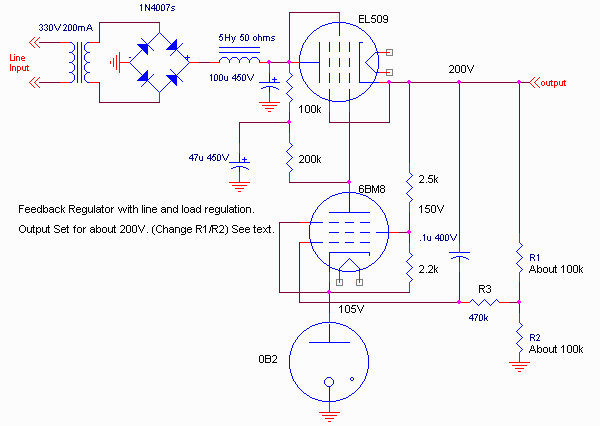This is a common circuit, that shows up with different part values in many places. I have altered the values to produce about 200 volts output. The output voltage is adjustable over a limited range by altering R1 and R2. In addition, the 0.1u capacitor from the output to the error amplifier grid has two purposes. First, it provides more error injection of the hum components, as mentioned above, to provide better ripple rejection. The second purpose is to aid in stability of the feedback circuit. Sometimes you will also see a small capacitor across the 0B2 VR tube. Its purpose is to remove any high frequency noise generated by the VR tube. DO NOT EVER use a large capacitor across these tubes. When used, a 0.01uF or so is about as large as you should use, although I've seen this same circuit with a 0.1uF across the VR tube.

This circuit provides pretty good regulation.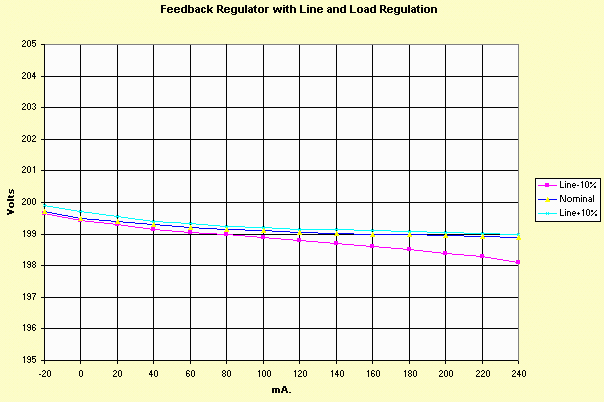Note that since this circuit provides even better regulation than those of part 2, I have again changed the scale! The ripple measured <1mV at 100mA output. It also has, as you might note in the above graph, the ability to SINK current as well as source current, which keeps any potential transients coming from an output stage, for instance, from causing the regulator to "un-regulate".

There are a couple deficiencies with this circuit, though. By placing the reference on the error amplifier cathode, there is now a definite minimum voltage that can be achieved (a disadvantage if you wanted a universally adjustable regulated supply). The 105 volts on the cathode implies that the plate of the error amplifier can go down to about 130 volts. So the grid of the series pass tube can never go lower than 130 volts. This makes the practical lower limit of the output somewhere about 155 volts (allowing 25 volts to "cutoff" the series pass tube, for low currents). The upper limit is the supply voltage produced, the maximum desired current, and the voltage drop across the series pass tube. With the values shown, about 220 volts at 100mA is about the limit. Since the cathode of the error amplifier is elevated, both the series pass tube and the error amplifier tube need to have separate heater windings, to keep the heater to cathode voltage in range, particularly if a wide range variable supply is sought.

Also, the additional capacitors in the circuit, along with the feedback style regulation cause the output impedance to vary with frequency. If you take the average slope of the above regulation curve, the effective "DC" output resistance is about 1 to 1.5 ohms. Here's the AC impedance vs frequency: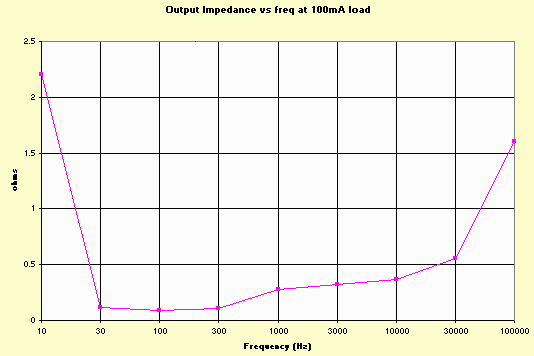Some of the deficiencies of this circuit are relatively easy to fix, however. We could provide a -150 volt reference (which could then be used for bias purposes), and instead of grounding the bottom of R2, we could connect it to -150 volts. We could then ground the cathode of the error amplifier. This actually allows better regulation, since the error amplifier cathode impedance is even lower, and the voltage division ratio turns out to be more favorable.

This style regulator looks line: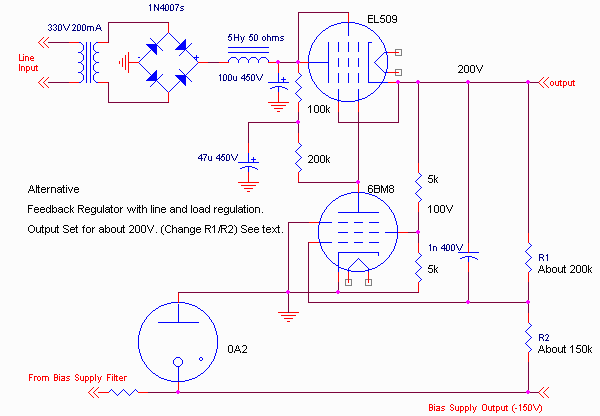This style regulator had virtually identical regulation performance to the one above, and about the same ripple characteristics. Basically the lower cathode impedance at the error amplifier (ground) offset the slightly different voltage divider action of the grid. I also change the 0.1uF capacitor to 1nF. This provides a more uniform output impedance over the audio frequency range (about 0.4 ohms). Since the cathode of the error amplifier is grounded, there is no problem finding a source for the heater voltage. Of course, you need to provide a negative supply for the 0A2, but this is realistically probably necessary for bias purposes anyway. The screen dropping resistors change, but the current is about the same, so this supply also had the same "sink" current capability.

The other advantage of this circuit is the ability to provide lower output voltages if needed, so it is much more flexible when considering a regulator providing a variable output for "experimental" purposes.

Error Amplifier Tube Characteristics

What makes a good error amplifier tube? From the above discussion, these characteristics become fairly obvious. For higher voltage applications, the error amplifier "sees" essentially the entire output voltage, so a high voltage device is needed. The current and power ratings are not usually too important; note that in the above circuits, there is not much power nor current going THROUGH the error amplifier. A high gain is desirable. This is why the typical error amplifier tube is a "high voltage" pentode. This is also why I used a 6BM8 as the above example. The high gain and high voltage capabilities are quite nice. (It also has an additional triode section which we will use in part 4 of this series).

A 6SN7 would work, for those who MUST use a triode, but you would probably need to place it in a cascode configuration in order to achieve sufficient gain. The limitation would then be the minimum upper plate to lower cathode voltage differential limiting the MINIMUM voltage.

A Practical Example

It's time to put the fundamentals we have been building in this series to a practical example. Lets consider building a "universal" regulator that is capable of providing a regulated output in the range of 50 to 450 volts, with a few hundred mA current range over most of the supply voltage range. We want the output resistance to be in the ballpark of one ohm (0.2V change for 200mA current change), and we want the power supply output ripple to be in the range of 1mV or less. We will also provide a fixed negative supply of 150 volts for bias.

For the main power transformer, I will consider the BFT-1, with additional ancillary transformers as necessary to cover the voltage range and other requirements as needed. (Caution I don't own a BFT-1, so I'm using transformers I have that I believe should perform the same, but there's still the off chance I messed up). I will break the schematic into two portions; the first is the transformers, rectifiers and filters, the second portion is the regulation circuit.

Here's the transformers, rectifiers and filters: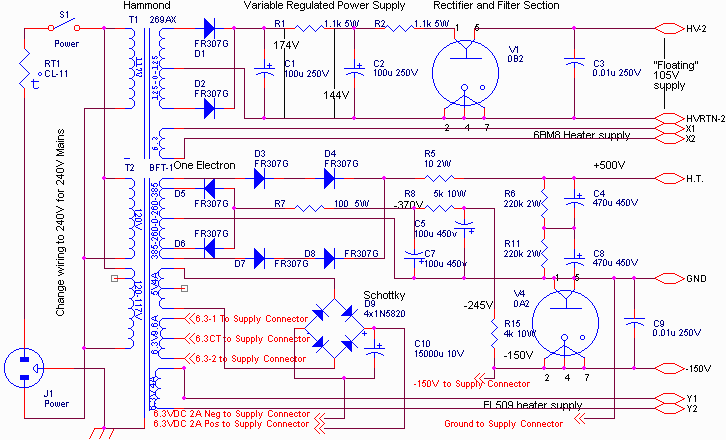And here is the regulator: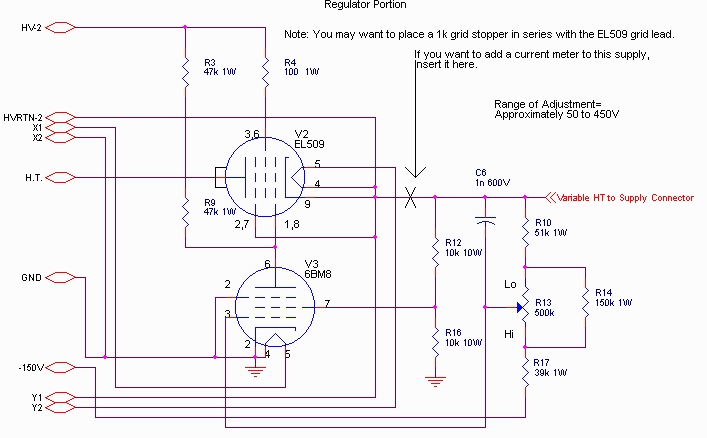The range of adjustment with this supply is from about 50volts to about 450 volts at nominal line and 50 volts to 410 volts with a 10% low line. (Due to the dropout voltage of the regulator.) The low voltage limit is due to the screen voltage placed on the error amplifier (25 volts at 50 volts output).

I have provided a number of things with this regulator:

1. An isolated 6.3VAC 9.6A AC heater supply for your experimental amp.
2. An isolated 6.3VDC at 2A max heater supply. This is filtered but not regulated.
3. A -150V at about 15mA max bias source. This is regulated and has about 200 microvolts of ripple.
4. A variable regulated supply with about 0.7 ohm output impedance, relatively flat across the audio band. This supply is variable from about 50V to about 450 volts. Hum level is less than 1mV at any valid output condition. (See table below). Typical hum level is about 250 microvolts, even with no choke in the supply. I will caution you, though, that to achieve this hum level, you have to be careful about your grounding. The high ripple currents from the filter caps should not be "in series with" the error amplifier in finding a ground. The schematic sort of suggests the order of grounding things.
5. There is some current sinking ability with this supply as discussed above.

The maximum current available from the supply is a function of the output voltage you set. At high output voltages, the output current is limited by the dropout voltage (I measure about 30 volts at 150mA), and at low output voltages by the power dissipation of the tube. Reasonable limits are:

 Output Voltage Maximum Current 50 50mA 100 80mA 150 100mA 200 120mA 250 150mA 300 210mA 350 350mA 400 250mA 450 125mA

Here is typical regulation characteristics (measured at 350 volts output).Notice that the performance is just slightly better than the previous example: the DC output resistance of this supply is only about 0.7 ohm!

An Interesting Tweak

If you connect a resistor of about 850k from the EL509 plate to the 6MB8 screen grid, then as the load current increases (causing the plate voltage of the EL509 to decrease), then you add additional compensation. For DC conditions, if you adjust the value of this resistor, you can achieve essentially perfect compensation: the load voltage REMAINS THE SAME regardless of the load! However, this is true of static conditions only. Here is one case where the DC output resistance is zero, but the AC output impedance is not zero. Looks fine on "specs", but, to my ears anyway, doesn't sound good, and looking at the output of the supply, feeding an amplifier shows some less-than-desireable signal. I mention this as a caution against blindly following specs, without really understanding the meaning behind them.

Can we do even better than this?

-Steve